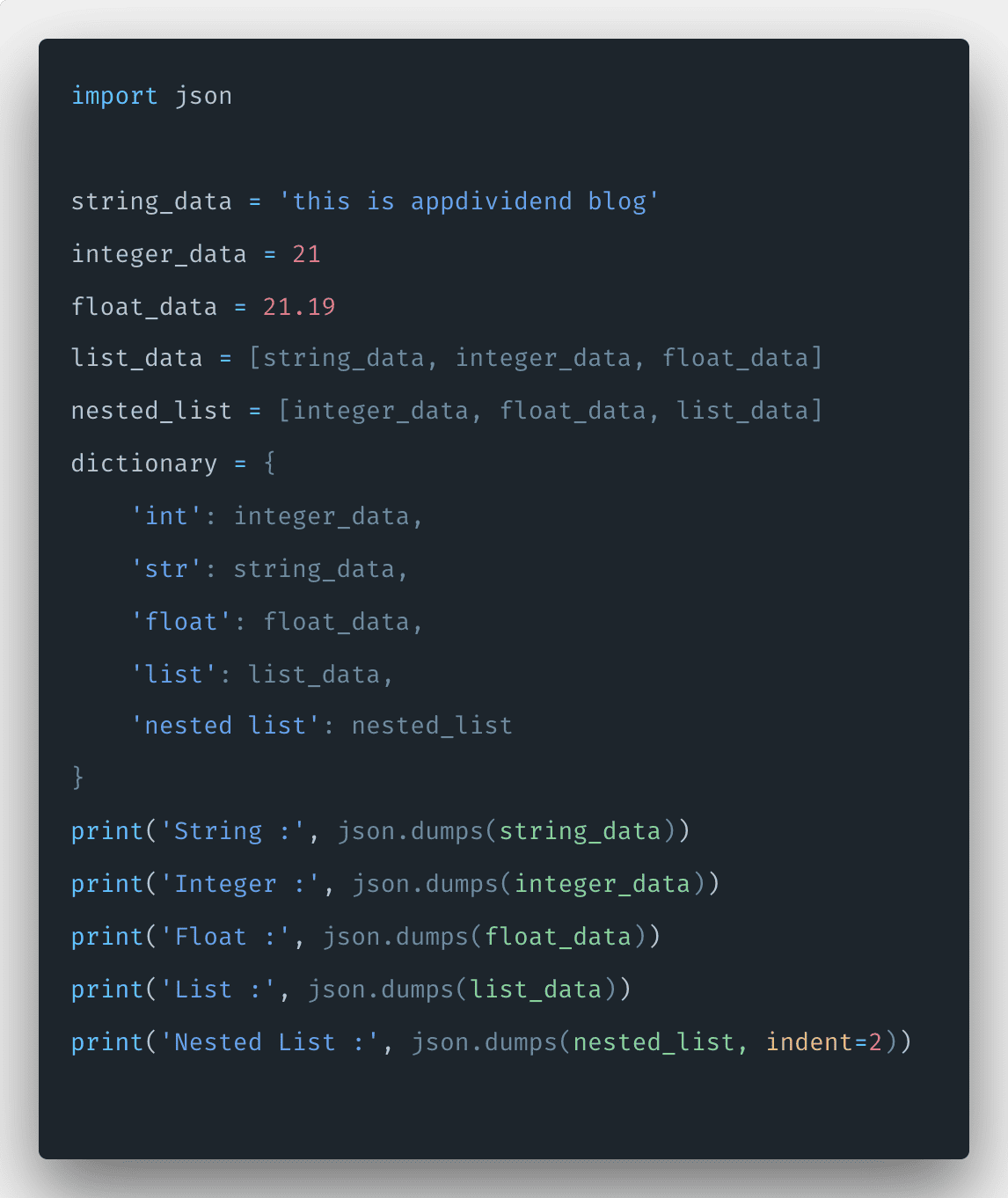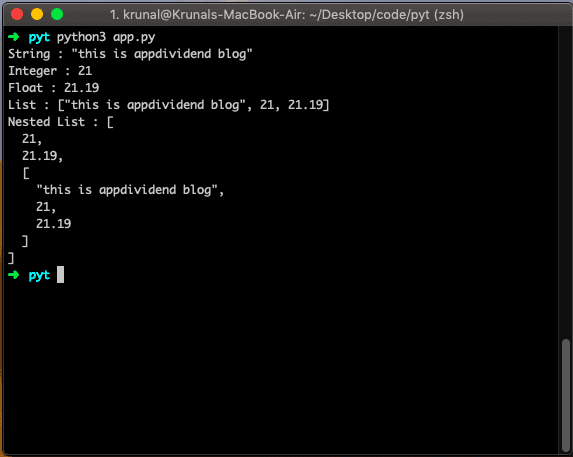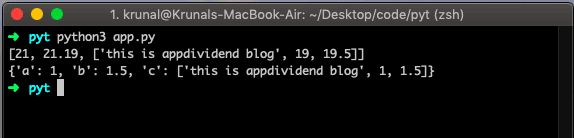# Python JSON parse: How To Parse JSON in Python

0
58JSON stands for JavaScript Object Notation, is a text-based format that facilitates data interchange between diverse applications. For example, an application written in ASP.NET running on Windows Server can easily exchange JSON data with an application written in Python and running on Linux.

Python provides the json module, which can be imported to any file and use to both parse JSON, as well as generate JSON from python objects and lists.

The most common JSON entity that you will encounter is an object: a set of key-value mappings in the format shown below.

```{
"firstName": "Krunal",
"lastName": "Lathiya",
"education": "IT Engineer",
"age": 25
}```

Here is how you can represent an array of objects.

In this representation, each item of the array is an object. JSON is a popular data format used for asynchronous browser/server communication. You can identify JSON using the following key points.

1. The data is only a name-value pair.
2. Data/Objects/arrays are separated by the comma.
3. Curly braces hold an object.
4. Square holds the array.

## Python json.dumps()

To convert any data to json format, use the json.dumps() method. The json.dumps() method converts a Python object into a json string.

Let us take almost all type of data in the example and convert into JSON and print in the console.

```# app.py

import json

string_data = 'this is appdividend blog'
integer_data = 21
float_data = 21.19
list_data = [string_data, integer_data, float_data]
nested_list = [integer_data, float_data, list_data]
dictionary = {
'int': integer_data,
'str': string_data,
'float': float_data,
'list': list_data,
'nested list': nested_list
}
print('String :', json.dumps(string_data))
print('Integer :', json.dumps(integer_data))
print('Float :', json.dumps(float_data))
print('List :', json.dumps(list_data))
print('Nested List :', json.dumps(nested_list, indent=2))```

See the output below.## How to Parse JSON data in Python

To parse json data in Python, use the json.loads() method. By using json.loads() function, you can convert JSON data into Python data. You can easily parse JSON data to Python objects.

Let see the following example.

```# app.py

import json

arrayJson = '[21, 21.19, ["this is appdividend blog", 19, 19.5]]'
objectJson = '{"a":1, "b":1.5 , "c":["this is appdividend blog", 1, 1.5]}'